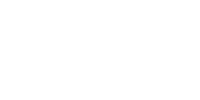Learning Python: Basic Mathematical Operators - Deepstash# Learning Python: Basic Mathematical Operators

For todays part we, are looking at basic numerical operators. In the image i have created 2 integer variables and then in the print i have used both variables and then used the operator inbetween them, so to add them we use '+', to subtract '-', mulyply '*' and finally divide '/'.

As you can see by the outputs it has worked, so 10 + 5 = 15, 10/5 = 2. These are basic operators for python, you can perform squaring and so on but thats for future content.

Next lesson will be about what happens if you added a string and integer together.

Dont forget to join Discord for help.

3 STASHED

1 LIKE

Deepstash helps you become inspired, wiser and productive, through bite-sized ideas from the best articles, books and videos out there.

GET THE APP:RELATED IDEASIn this part we will be going over more on variables and printing them.

So let's make a variable and give it the value of 10, so it should look something like this X = 10, X could be any word really just not a reserved word, here is a link to a website with alot of reserved words that can't be used https://realpython.com/lessons/reserved-keywords/.

and scroll down a little and you will see key words.

Once you have the variable made let's print the variable.

So like before very simply print(x), as you can see in the image above. Now lets run and se the output which in this case be 10.

7 STASHED

3 LIKESJust like last week i have created a for loop, but instead of only one number in the brackets.

The first number is the starting value and the second value is the highest, so in this case i have put 10, 100 so the range is 10 to 99, the last number which i put as 5, is the steps, so every 5 numbers it will do what is in the for loop. This could be any number range, plus it can go the other way aswell, so when you make the for loop in the pair of brackets put 100, 0 , -1 and it will count down instead.

For loops can also be used to iterate through lists, but that is the next lesson

Join discord

3 STASHED

1 LIKEThe first thing we need to consider when starting to learn a programming language, is what shall we use. There are a few things we can use, for example python IDLE, pycharm, VS code, notepad++, anaconda or Google Colab.

For these lessons I would recommend pycharm. But first we need to install python.